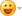# test

```/***********************************************************************
Little PCI Core
data: 2000/10/10
***********************************************************************/
`define  VID   16'H1234
`define  DID   16'HABCD

module pci (
// PCI ports -- do not modify names!
AD,
CBE,
//     PAR,
FRAME_N,
TRDY_N,
IRDY_N,
STOP_N,
DEVSEL_N,
IDSEL,
RST_N,
PCLK,
INTR_N,     //PCI Interrupt output Low level active
//LOCAL Signals
RD_EN,
WR_EN,
ADDR,
P_L_DA,
L_P_DA,
INT      //local Interrupt, high level active
);

inout[31:0]AD;
input   [3:0]CBE; //inout   [3:0] CBE;
input        FRAME_N; //inout        FRAME_N;
output        TRDY_N;
inputIRDY_N;
output        STOP_N;
output         DEVSEL_N;
input         IDSEL;
input         RST_N;
input         PCLK;
output         INTR_N;

output         RD_EN;
output        WR_EN;
output [4:0]   ADDR;
input  [31:0]  L_P_DA;
output [31:0]  P_L_DA;
input          INT;

`define state parameter
`state    S_IDLE_S = 2'B00;
`state    S_BUSY_S = 2'B01;
`state    S_DATA_S = 2'B10;
`state    S_STOP_S = 2'B11;

reg  [1:0]    current_state,next_state;
reg           CFG_EN;       //CFG_EN = 1 : PCI config read/write, CFG_EN = 0: PCI Mem Read/Write
reg           S_DATA_REG;
reg           HIT;
reg           S_RD,S_WR;
reg [11:0]    BAR0_REG;     //{BAR0_REG,20'h00}: 1M PCI MEM Space,last 20 bit is hardware as 0
reg  [4:0]    ADDR;
reg           S_DATA;
reg [31:0]    AD_D;
wire          TRDY,STOP;
//State Machine
always @(posedge PCLK or negedge RST_N)
begin
if (~RST_N) current_state <= 2'b0;
else  current_state <= next_state;
end
always @(FRAME_N or IRDY_N or HIT or TRDY or STOP or IRDY_N or current_state )
begin
next_state = current_state;
case (current_state)
S_IDLE_S: if (!FRAME_N & !HIT) next_state = S_BUSY_S;
S_BUSY_S: begin
if (FRAME_N & !HIT) next_state = S_IDLE_S;
else if ((!FRAME_N || !IRDY_N) & HIT) next_state = S_DATA_S;
end
S_DATA_S: if (FRAME_N & !TRDY) next_state = S_IDLE_S;
else if (~STOP) next_state = S_STOP_S;
S_STOP_S: if (FRAME_N) next_state = S_IDLE_S;
default: next_state = S_IDLE_S;
endcase
end

//address compare with BAR0
wire #1 AD_EQ = (AD[31:20] == BAR0_REG);   //BAR0 Support 1M MEM Space (but littlr PCI CORE only support
//                            5bit (32byte) Mem Space)
always @(posedge PCLK or negedge RST_N)
begin
if (~RST_N) begin
S_WR <= 1'B0;
S_RD <= 1'B0;
CFG_EN <= 1'B0;
HIT <= 1'B0;  //BASE HIT, CFG HIT
ADDR <= 5'H0;
end
else if (!FRAME_N && (current_state == S_IDLE_S)) begin
ADDR <= AD[4:0];        //littlr PCI CORE only support 5bit (32byte) Mem Space
casex ({CBE,IDSEL})
5'B0110X: begin
S_WR <= 1'B0;
S_RD <= 1'B1;  //PCI MEM Read
CFG_EN <= 1'B0;
if (AD_EQ) begin
HIT <= 1'B1;
end
end
5'B0111X: begin
CFG_EN <= 1'B0;
S_WR <= 1'B1;  //PCI MEM Write
S_RD <= 1'B0;
if (AD_EQ) HIT <= 1'B1;
end
5'B10101: begin
CFG_EN <= 1'B1;
S_RD <= 1'B1;    //PCI CFG Read
S_WR <= 1'B0;
HIT <= 1'B1;
end
5'B10111: begin
CFG_EN <= 1'B1;
S_RD <= 1'B0;    //PCI CFG Write
S_WR <= 1'B1;
HIT <= 1'B1;
end
default: begin
S_WR <= 1'B0;
S_RD <= 1'B0;
HIT <= 1'B0;
end
endcase
end
else if (current_state == S_BUSY_S)
HIT <= 1'B0;
end

//S_DATA: is register output, in order to mach CLK to PAD delay <7.8ns
always @(posedge PCLK or negedge RST_N)
begin
if (~RST_N) S_DATA <= 1'B0;
else S_DATA <= (next_state == S_DATA_S);
end
always @(posedge PCLK or negedge RST_N)
begin
if (~RST_N) S_DATA_REG <= 1'B0;
else S_DATA_REG <= S_DATA;
end
//TAR_DLY: delete 1 clock
wire #1 TAR_DLY = S_DATA_REG;
//Address Decode
wire #1 ad_00 = (ADDR == 5'h00);
wire #1 ad_10 = (ADDR == 5'h10);
wire #1 we_en = S_DATA & S_WR;
wire #1 rd_en = S_DATA & S_RD;
wire S_TERM = 1'B0;
wire S_READY = 1'B1;

wire #1 RD_WR = S_DATA & (S_WR || (S_RD & TAR_DLY));
//assign #1 TRDY = !(RD_WR & (CFG_EN || S_READY));
assign #1 TRDY = ~RD_WR;  //a optimize , add at 15/01/2002
//assign #1 STOP = ~(RD_WR & (CFG_EN || S_TERM));//1'B1;
assign #1 STOP = ~(RD_WR & CFG_EN);//a optimize , add at 15/01/2002
wire #1 OE = S_DATA || TAR_DLY;
wire #1 DEVSEL = ~S_DATA;
assign TRDY_N = OE ? TRDY : 1'BZ;       //tri gate output: is more slower
assign STOP_N = OE ? STOP : 1'BZ;
assign DEVSEL_N = OE ? DEVSEL : 1'BZ;
//CFG
//CFG Address Decode
wire #1 RD_ID   = rd_en &  CFG_EN & TAR_DLY & ad_00;//(ADDR == 5'h00);
wire #1 RD_BAR0 = rd_en &  CFG_EN & TAR_DLY & ad_10;//(ADDR == 5'h10);
wire #1 WE_BAR0 = we_en &  CFG_EN & ad_10;//(ADDR == 5'h10);
//CFG Write : BAR0
always @(posedge PCLK or negedge RST_N)
begin
if (~RST_N) BAR0_REG <= 12'B0;
else if (WE_BAR0) BAR0_REG <= AD[31:20];//ADIO[31:20];
end

wire #1 WR_EN = we_en &  ~CFG_EN ;
wire #1 RD_EN = rd_en &  ~CFG_EN & TAR_DLY ;

//PCI read data
always @(RD_ID or RD_BAR0 or RD_REG or TEST_REG or BAR0_REG)
begin
case ({RD_ID,RD_BAR0,RD_REG})
3'b001: AD_D = {24'H0,TEST_REG};
3'B010: AD_D = {BAR0_REG,20'h0};
3'B100: AD_D = {`DID,`VID};
default:AD_D = 32'B00;
endcase
end
assign AD = rd_en ? AD_D : 32'BZZ;
assign P_L_DA = AD;
//PCI Interrupt Output
assign INTR_N = INT ? 1'B0 : 1'BZ;   //Tri gate output, need outside pullup res
endmodule```

## 全部作者的其他最新日志

### 评论 (0 个评论)• 关注TA
• 加好友
• 联系TA

周排名

总排名

关注

粉丝

好友

获赞

评论
• ### 6

收藏

GMT+8, 2021-4-20 08:02 , Processed in 0.048273 second(s), 24 queries .